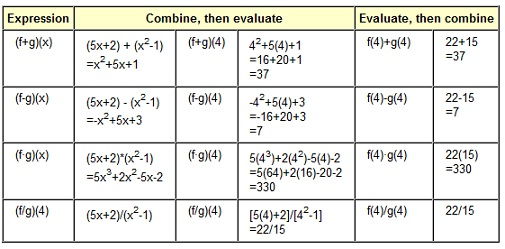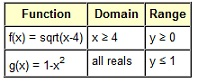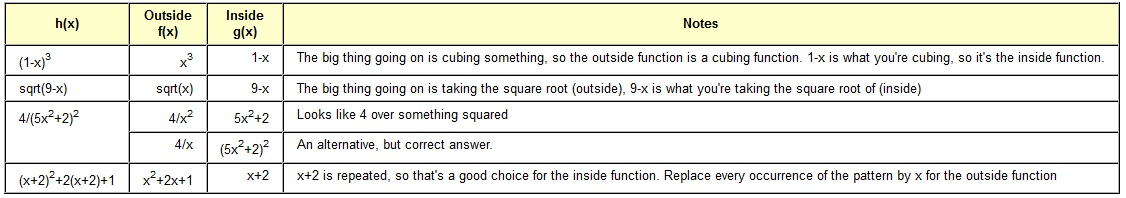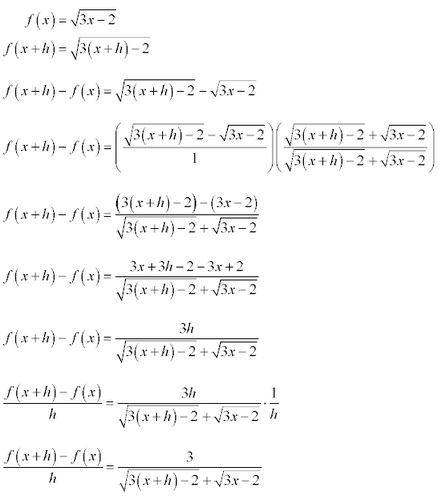#### Concept of Combinations of Functions

Combinations of Functions:

Arithmetic Combinations of Functions:

The difference, sum, product or quotient of functions can be found without difficulty.

Sum:

(f + g)(x) = f(x) + g(x)

Difference:

(f - g)(x) = f(x) - g(x)

Product:

(f • g)(x) = f(x) • g(x)

Quotient:

(f/g)(x) = f(x)/g(x), as long as g(x) is not zero.

The domain of each of such combinations is the intersection of domain of f and the domain of g. In another words, both the functions should be defined at a point for combination to be defined. One additional need for the division of functions is that the denominator cannot be zero; however we knew that as it is part of implied domain.

Fundamentally what the above states is that to calculate a combination of functions, you might join the functions and then calculate or you might calculate each function and then join.

Illustrations:

In the give below illustrations, let f(x) = 5x+2 and g(x) = x2-1. We will then compute each combination at point x = 4. f(4) = 5(4)+2 = 22 and g(4) = 42-1 = 15As you can see from illustrations, it does not matter if you join and then compute or if you compute and then join.

In each of the above troubles, the domain is all real numbers with the exception of division. Domain in the division combination is all real numbers apart from for 1 and -1.

Composition of Functions:

As the arithmetic combinations of functions are straightforward and quite simple, there is the other kind of combination termed as the composition.

The composition of functions is applying of one function to the other function. Symbol of composition of functions is a small circle among the function names. The symbol is in text mode on, therefore we use a lower case oh "o" to symbolize composition of functions.

(fog)(x) = f [g(x)]
(gof)(x) = g [f(x)]

These are read as "f composed with g of x" and "g composed with f of x" correspondingly.

The function on the outside is forever written first with the functions which follow being on inside. The order is significant. The composition of functions is not commutative.

Illustrations of Composition of Functions:

Some of the illustrations are as follows:

f(x) = 5x+2 and g(x) = x2-1

(fog)(x) = f [g(x)] = f [x2-1 ] = 5(x2-1 ) + 2 = 5x2- 5 + 2 = 5x2-3

(gof)(x) = g [f(x)] = g [5x+2] = (5x+2)2 - 1 = 25x2 + 20x + 4 - 1 = 25x2 + 20x + 3

f(x) = sqrt(x) and g(x) = 4x2

(fog)(x) = f [g(x)] = f [4x2] = sqrt(4x2) = 2 |x|

(gof)(x) = g [f(x)] = g [sqrt(x)] = 4 (sqrt(x))2 = 4x, x ≥ 0

This illustration probably needs some description. We know that the square root of (x2) is the absolute value of x. The square of (square root of x) is x, however this supposes that x is not negative as you could not find out the square root of x in the first place if it was. It is a case where the implied domain (as of square root) is no longer implied (as the square root is gone), therefore you have to explicitly state it.

f(x) = sqrt(x-4) and g(x) = 1 - x2

(fog)(x) = f [g(x)] = f [1-x2] = sqrt ([1-x2] - 4) = sqrt (-x2 - 3) = ø

(gof)(x) = g [f(x)] = g [sqrt(x-4)] = 1 - [sqrt(x-4)]2 = 1 - (x-4) = 5 - x, x ≥ 4

If the last illustration required some description, then this one definitely requires some, too. Let us take the simpler one (gof)(x) first. There was an implied domain of x ≥ 4 since of the square root, however after squaring it, it was no longer implied, and therefore it is needed to be stated explicitly.

Now for the tougher one (fog)(x). Here the simple explanation is described first and the more complete one later. After simplifying, you got the square root of (-x2 - 3). -x2-3 is always negative, no matter what real number x is, and you cannot take the square root of negative number, therefore it is always undefined for the set of real.

Determining Domains on Composition of Functions:

Whenever you find a composition of the functions, it is no longer x which is being plugged to the outer function, it is the inner function computed at x. Therefore there are two domains which we have to be concerned about. When we consider (fog)(x), we see that g is computed at x, therefore x has to be in the domain of g. We as well see that f is computed at g(x), therefore g(x) has to be in domain of f.

a) For (fog)(x), x is the value which can be plugged to g and gives you the value g(x) which can be plugged to f to get the f(g(x)).

b) For (gof)(x), x is the value which can be plugged to f and gives you the value f(x) which can be plugged to g to get the g(f(x)).

Let us consider that last illustration again.Whenever you find (fog)(x), there are two things which should be satisfied:

A) x should be in the domain of g that means x is the real number (pretty simple to do).

B) g(x) should be in the domain of f, that signifies that 1-x2^2 ≥ 4 (whenever you try to solve this, you obtain the empty set).

Whenever you join the two domains to see what they have in common, you find out the intersection of everything and nothing is anything (that is, the empty set), therefore the function is stated nowhere and undefined everywhere.

Whenever you find out (gof)(x), there are two things which should be satisfied:

a) x should be in the domain of f, that signifies that x ≥ 4 (not too bad).

b) f(x) should be in the domain of g that signifies that sqrt(x-4) should be a real number (which takes place when x ≥ 4).

Whenever you join the two domains to see what they have in common, you find out the intersection to be x ≥ 4, and hence the composition is defined.

Decomposition of Functions:

The decomposition of functions is reverse of composition of functions. Rather than joining two functions to obtain a new function, you are breaking apart a joined function to its separate components. There is frequently more than one way to decompose a function; therefore your answers might differ from the books.

Fundamentally, you wish to look at the function and look for an ‘outside function’ and an ‘inside function’. The other thing to look for is repeated patterns and make the inside function. The outside function is concluded as ‘the big picture’ and the inside function is ‘what you are doing to big picture to’.

Illustrations:

Write each and every function h as the composition of two functions f and g in such a way that h(x) = (fog)(x)Difference Quotients:

Difference quotients are what they state they are. They include a difference and a quotient. The difference quotient is actually the slope of a secant line among the two points on a curve.

The formula for difference quotient is: [f(x+h) - f(x)]/h

When you go to calculus, you will be introduced to the concept of limits (that is, Calculus is Algebra with limits) and find out as the two points on the curve get nearer together, the secant line turns to a tangent line, and the slope of secant line becomes the slope of tangent line that is termed as the derivative of function and there are a lot of shortcuts for finding out derivatives. The difference quotient is much important in Calculus, therefore if you are going on, make sure you acquire this!

For polynomial functions, finding out the difference quotient is not that difficult. Where you are going to run to trouble is with radical and rational functions.

Polynomial Functions:

f(x) = 5x2 - 2
f(x+h) = 5(x+h)2 - 2 = 5( x2 + 2xh + h2 ) - 2 = 5x2 + 10xh + 5h2 - 2
f(x+h) - f(x) = 5( x2 + 2xh + h2 ) - 2 = 5x2 + 10xh + 5h2 - 2 - ( 5x2 - 2 )
f(x+h) - f(x) = 5x2 + 10xh + 5h2 - 2 - 5x2 + 2 = 10xh + 5h2
f(x+h) - f(x) = h (10x + 5h)
[f(x+h) - f(x)]/h = h (10x + 5h)/h = 10x + 5h

Rational Functions:

The trick with rational functions is to obtain a common denominator and then simplify it.

f(x) = 3/x
f(x+h) = 3/(x+h)
f(x+h) - f(x) = 3/(x+h) - 3/x
f(x+h) - f(x) = 3 x/[ x(x+h)] - 3(x+h)/[x(x+h)]
f(x+h) - f(x) = (3x - 3x - 3h)/[x(x+h)]
f(x+h) - f(x) = -3h / [x(x+h)]
[f(x+h) - f(x)]/h = -3h/[x(x+h)]/h = -3/[ x(x+h)]